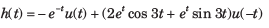Courses

# The Laplace Transform - MCQ Test

## 20 Questions MCQ Test RRB JE for Electronics & Communication Engineering | The Laplace Transform - MCQ Test

Description
This mock test of The Laplace Transform - MCQ Test for Railways helps you for every Railways entrance exam. This contains 20 Multiple Choice Questions for Railways The Laplace Transform - MCQ Test (mcq) to study with solutions a complete question bank. The solved questions answers in this The Laplace Transform - MCQ Test quiz give you a good mix of easy questions and tough questions. Railways students definitely take this The Laplace Transform - MCQ Test exercise for a better result in the exam. You can find other The Laplace Transform - MCQ Test extra questions, long questions & short questions for Railways on EduRev as well by searching above.
QUESTION: 1

### Given the transform pair below. Determine the time signal y(t) and choose correct option.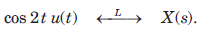​ ​ ​ Que: Y(s) = (s + 1)X(s)

Solution: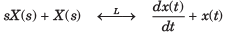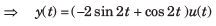QUESTION: 2

### Given the transform pair below. Determine the time signal y(t) and choose correct option.Que: Y(s) = X(3s)

Solution: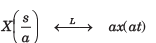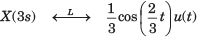QUESTION: 3

### Given the transform pair below. Determine the time signal y(t) and choose correct option.Que: Y(s) = X(s + 2)

Solution: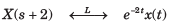QUESTION: 4

Unilateral Laplace Transform is applicable for the determination of linear constant coefficient differential equations with ________

Solution:
QUESTION: 5

Given the transform pair below. Determine the time signal y(t) and choose correct option.Que: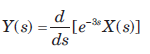Solution: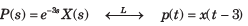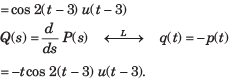QUESTION: 6

Given the transform pair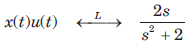Determine the Laplace transform Y(s) of the given time signal in question and choose correct option.

Que: y(t) = x(t - 2)

Solution: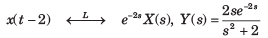QUESTION: 7

Given the transform pairDetermine the Laplace transform Y(s) of the given time signal in question and choose correct option.

Que: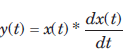Solution: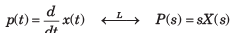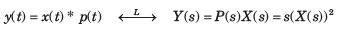QUESTION: 8

Given the transform pairDetermine the Laplace transform Y(s) of the given time signal in question and choose correct option.

Que: y(t) = e-t x(t)

Solution: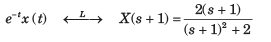QUESTION: 9

Given the transform pairDetermine the Laplace transform Y(s) of the given time signal in question and choose correct option.

Que: y(t) = 2tx(t)

Solution: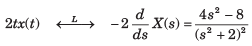QUESTION: 10

Determine the bilateral laplace transform and choose correct option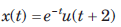​ ​ ​

Solution: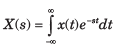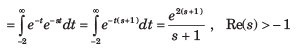QUESTION: 11

Determine the bilateral laplace transform and choose correct option.

x(t) = u(-t + 3)

Solution: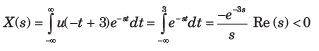QUESTION: 12

Determine the bilateral laplace transform and choose correct option

y(t) = δ(t + 1)

Solution: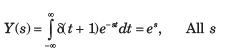QUESTION: 13

Determine the bilateral laplace transform and choose correct option

x(t) = sin t u(t)

Solution: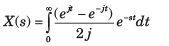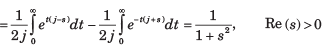QUESTION: 14

Determine the bilateral laplace transform and choose correct option.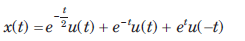Solution: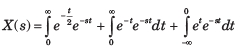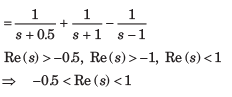QUESTION: 15

Determine the bilateral laplace transform and choose correct option.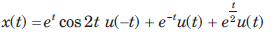Solution: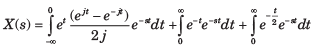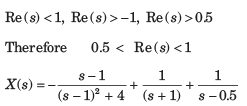QUESTION: 16

Determine the bilateral laplace transform and choose correct option.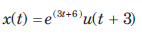​ ​ ​

Solution: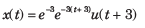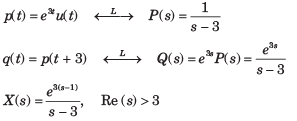QUESTION: 17

Where is the ROC defined or specified for the signals containing causal as well as anti-causal terms?

Solution:
QUESTION: 18

The transform function H(s) of a causal system is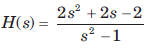The impulse response is

Solution:

For a causal system h(t) = 0 for t < 0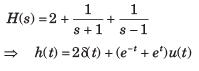QUESTION: 19

The transfer function H(s) of a stable system is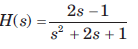The impulse response is

Solution: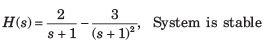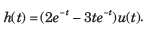QUESTION: 20

The transfer function H(s) of a stable system is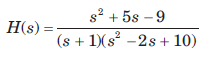The impulse response is

Solution: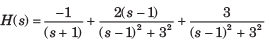System is stable